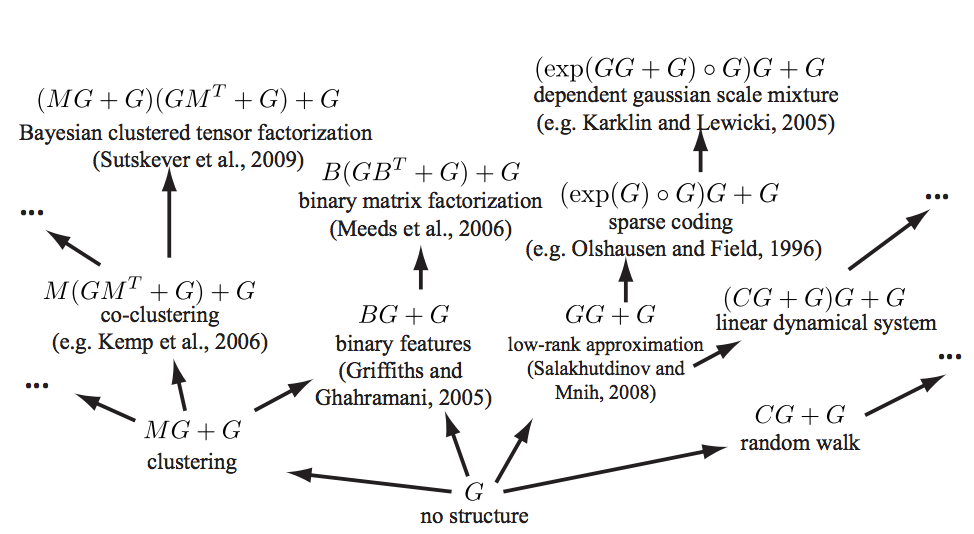# Combining kernelsA sum or product (or outer sum, or tensor product) of Mercer kernels is still a kernel. For other operations YMMV; let us look at some.

Since talking about kernels in the abstract is confusing, I like to consider a (slightly) more concrete mental model: Which kernels are valid covariance functions for a Gaussian process? This turns out to be a useful way to think about it because it suggests some ways to combine kernels.

## Operations preserving positivity

For example, in the case of Gaussian processes, suppose that, independently,

\begin{aligned} f_{1} &\sim \mathcal{GP}\left(\mu_{1}, k_{1}\right)\\ f_{2} &\sim \mathcal{GP}\left(\mu_{2}, k_{2}\right) \end{aligned} then $f_{1}+f_{2} \sim \mathcal{GP} \left(\mu_{1}+\mu_{2}, k_{1}+k_{2}\right)$ so $$k_{1}+k_{2}$$ is also a kernel.

More generally, if $$k_{1}$$ and $$k_{2}$$ are two kernels, and $$c_{1}$$, and $$c_{2}$$ are two positive real numbers, then $K(x, x')=c_{1} k_{1}(x, x')+c_{2} k_{2}(x, x')$ is again a kernel. What with the multiplication as well, we note that all polynomials of kernels where the coefficients are positive are in turn kernels .

Note that the additivity in terms of kernels is not the same as additivity in terms of induced feature spaces. The induced feature map of $$k_{1}+k_{2}$$ is their concatenation rather than their sum. Suppose $$\phi_{1}(x)$$ gives us the feature map of $$k_{1}$$ for $$x$$ and likewise $$\phi_{2}(x)$$. Then \begin{aligned} k_{1}(x, x') &=\phi_{1}(x)^{\top} \phi_{1}(x') \\ k_{2}(x, x') &=\phi_{2}(x)^{\top} \phi_{2}(x')\\ k_{1}(x, x')+k_{2}(x, x') &=\phi_{1}(x)^{\top} \phi_{1}(x')+\phi_{2}(x)^{\top} \phi_{2}(x')\\ &=\left[\begin{array}{c}{\phi_{1}(x)} \\ {\phi_{2}(x)}\end{array}\right]^{\top} \left[\begin{array}{c}{\phi_{1}(x')} \\ {\phi_{2}(x')}\end{array}\right] \end{aligned}

If $$k:\mathcal{Y}\times\mathcal{Y}\to\mathbb{R}$$ is a kernel and $$\psi: \mathcal{X}\to\mathcal{Y}$$ this is also a kernel

\begin{aligned} k_{\psi}:&\mathcal{X}\times\mathcal{X}\to\mathbb{R}\\ & (x,x')\mapsto k_{y}(\psi(x), \psi(x')) \end{aligned}

which apparently is now called a deep kernel. if $$k$$ is stationary and $$\psi$$ is invertible then this is a stationary reducible kernel.

Also if $$A$$ is a positive definite operator, then of course it defines a kernel $$k_A(x,x'):=x^{\top}Ax'$$

Genton (2001) uses the interpretation as covariance to construct some other useful combinations.

Let $$h:\mathcal{X}\to\mathbb{R}^{+}$$ have minimum at 0. Then, using the identity for RVs $\mathop{\textrm{Cov}}\left(Y_{1}, Y_{2}\right)=\left[\mathop{\textrm{Var}}\left(Y_{1}+Y_{2}\right)-\mathop{\textrm{Var}}\left(Y_{1}-Y_{2}\right)\right] / 4$

we find that the following is a kernel

$K(x, x')=\frac{1}{4}[h(x+x')-h(x-x')]$

All these to various cunning combination strategies, which I may return to discuss. 🏗 Some of them are in the references. For example Duvenaud et al. (2013) position their work in the wider field:

There is a large body of work attempting to construct a rich kernel through a weighted sum of base kernels . While these approaches find the optimal solution in polynomial time, speed comes at a cost: the component kernels, as well as their hyperparameters, must be specified in advance […]

Hinton and Salakhutdinov (2008) use a deep neural network to learn an embedding; this is a flexible approach to kernel learning but relies upon finding structure in the input density, $$p(x)$$. Instead we focus on domains where most of the interesting structure is in $$f(x)$$.

Wilson and Adams (2013) derive kernels of the form $$\operatorname{SE} \times \cos(x − x_0$$), forming a basis for stationary kernels. These kernels share similarities with $$\operatorname{SE} \times \operatorname{Per}$$ but can express negative prior correlation, and could usefully be included in our grammar.

See Grosse et al. (2012) for a mind-melting compositional matrix factorization diagram, constructing a search over hierarchical kernel decompositions.“Exploiting compositionality to explore a large space of model structures” indeed.

Examples of existing machine learning models which fall under our framework. Arrows represent models reachable using a single production rule. Only a small fraction of the 2496 models reachable within 3 steps are shown, and not all possible arrows are shown.

Here is another list of kernel combinations that preserve positive-definiteness, but for which I have tragically lost the source (it is one of the papers here).

\begin{aligned} K(x, y) &=K_{1}(x, y)+K_{2}(x, y) \\ K(x, y) &=c K_{1}(x, y)+K_{2}(x, y) \text { for } c \in \mathbb{R}_{+} \\ K(x, y) &=K(x, y)+c \text { for } c \in \mathbb{R}_{+} \\ K(x, y) &=K_{1}(x, y) K_{2}(x, y) \\ K(x, y) &=f(x) f(y) \text { for } f: \mathcal{X} \to \mathbb{R} \\ K(x, y) &=\left(K_{1}(x, y)+c\right)^{d} \text { for } c \in \mathbb{R}_{+} \text {and } d \in \mathbb{N} \\ K(x, y) &=\exp \left(K_{1}(x, y) / \sigma^{2}\right) \text { for } \sigma \in \mathbb{R} \\ K(x, y) &=\exp \left(-\left(K(x, x)-2 K(x, y)+K(y, y)\right) / 2 \sigma^{2}\right) \\ K(x, y) &=K_{1}(x, y) / \sqrt{K_{1}(x, x) K_{1}(y, y)} \end{aligned}

## Applying kernels over different axes

I silently assumed this works because it seems obvious sometimes; but then sometimes I forget this trick, so maybe it is not obvious:

For multi-dimensional inputs we can apply kernels over any subset of the axes, and thence different kernels over different subsets of the axes.

## Locally stationary kernels

Genton (2001) defines locally stationary kernels to mean those that have a particular structure, specifically, a kernel that can be factored into a stationary kernel $$K_2$$ and a non negative function $$K_1$$ in the following way:

$K(\mathbf{s}, \mathbf{t})=K_{1}\left(\frac{\mathbf{s}+\mathbf{t}}{2}\right) K_{2}(\mathbf{s}-\mathbf{t})$

Local structure then depends on the global mean location $$\frac{\mathbf{s}+\mathbf{t}}{2}$$. See the paper for some useful spectral properties of these kernels.

Other constructions might vie for the title of “locally stationary”. To check. 🏗

## Stationary reducible kernels

See kernel warping.

## Other nonstationary kernels

### No comments yet. Why not leave one?

GitHub-flavored Markdown & a sane subset of HTML is supported.